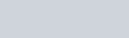In this video I show you how to use mathematical induction to prove the sum of the series

#### Prove the following:

1.#### The method of induction:

• Start by proving that it is true for n=1, then assume true for n=k and prove that it is true for n=k+1. If so it must be true for all positive integer values of n.